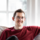# Compounding Values with Dynamic Multipliers

Coming from the world of spreadsheets, you may have defined a set of values recursively with ease, referencing the previous value plus another variable to calculate a new value. Something like this: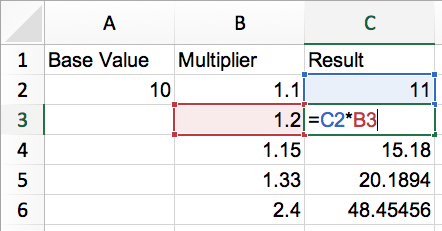In the above screenshot, the result column is calculated by multiplying the "Multiplier" by the previous result. This is called recursion and is a very powerful concept.

*Note: C2 is computed non-recursively. We call this the 'base case'

You may be wondering how you can do this in Periscope. It's super easy in Excel/Google Sheets, but what about SQL? If you're running a Postgres database, this would be possible with Recursive CTEs but on AWS Redshift, we don't have that ability.

The solution in Periscope is to use a scripting language such as Python or R! In this community post, I'll share and explain a solution in Python 3.6!

First, let's generate a dataset to practice on via SQL:

```select
10 as base_value
, 1.1 as multiplier
, 11 as expected_result
union all
select
null
, 1.2
, 13.2
union all
select
null
, 1.15
, 15.18
union all
select
null
, 1.33
, 20.1894
union all
select
null
, 2.4
, 48.45456```

This will give 3 columns: a base value, multipliers, and answers that I calculated in Excel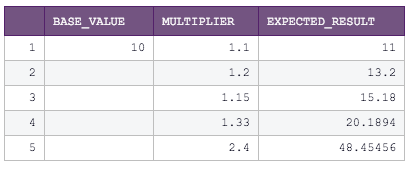Let's take a look at the finished code, then we'll explore how it works:

```# SQL output is imported as a pandas dataframe variable called "df"
import pandas as pd
import numpy as np

# Start of function definition
def compounder(df, x, y):
results = []
prev_val = df[x]

for (base_value, multiplier) in zip(df[x], df[y]):
if np.isnan(multiplier):
result = np.nan
results.append(result)
prev_val = base_value
else:
result = prev_val * multiplier
results.append(result)
prev_val = result

return pd.Series(results)
# End of function definition

df["calculated"] = compounder(df, "base_value", "multiplier")

# Output data to periscope
periscope.output(df)```

When running this, we get the following result: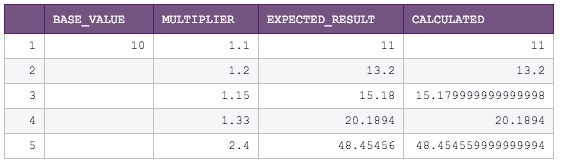Now we can compare the expected result to the calculated result and see that it's right on! Remember, the expected_result column here was manually added. With your actual data, this luxury probably will not be available so easily. We may wish to round the values, in which case we can add the round method to the return line of the function.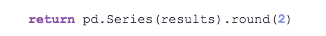We've got our answer, now let's step through how it works!

1. First we import both the pandas library and the numpy library to enable working with dataframes and with null values (which are known as NaN in Python, aka "Not a Number").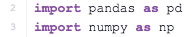2. Next, we define a function called "compounder" which takes a dataframe object, and the names of two columns we care about.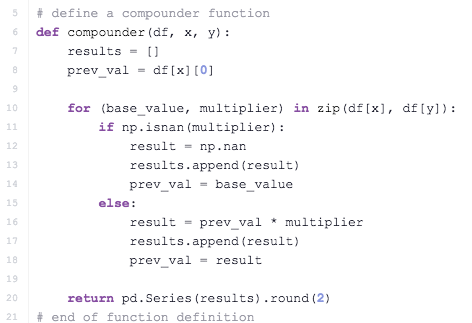3. Third, we declare a new dataframe column simply by assigning it the value generated by running the compounder function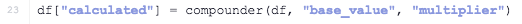4. Lastly, we output the dataframe to Periscope so it can be interpreted as a table and charted appropriately!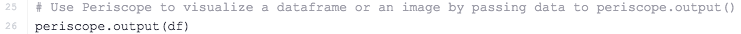The function definition is the most complex part. Within a for loop, it conditionally checks if a multiplier value exists, and calculates a computed value to add to the list which will be used to create the new column. Try it out for yourself!!

Bonus: For any advanced Python programmers out there, can you come up with a truly recursive function (a function that calls itself) that can be used to achieve the same result as this one?